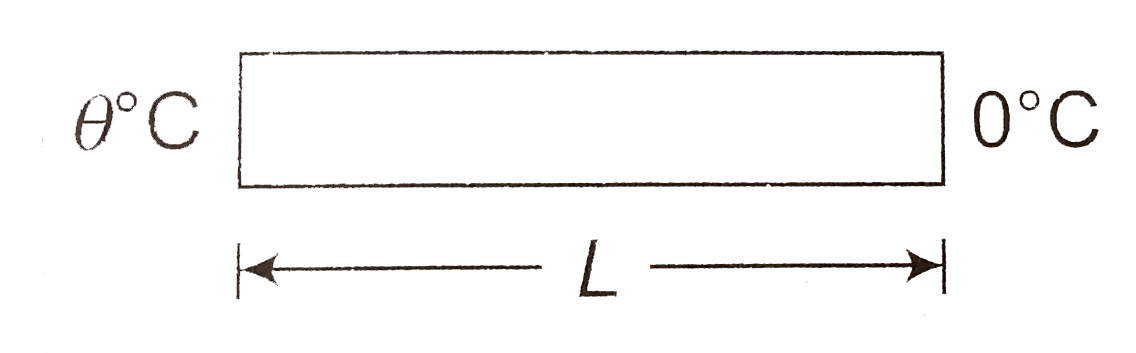# A rod of negligible heat capacity has length L, area of cross-section A and thermal conductivity K. The temperature of one end is maintained at

47 views
in Physics
closed
A rod of negligible heat capacity has length L, area of cross-section A and thermal conductivity K. The temperature of one end is maintained at 0^(@)C and the other end is slowly linearly varied from 0^(@)C to theta_(0)^(@)C in time t_(0) Assuming no loss of heat through the sides, find the total heat transimitted through the rod in time t_(0)

by (90.1k points)

Resistance of rod R = (L)/(KA)
The temperature of left end varies linearly from 0^(@)C to theta_(0).^(@)C in the time t_(0)
Temperature of left end at time t
theta = 0 + (theta_(0) - 0)/(t_(0)) t = (theta_(0))/(t_(0)) t
Heat current i = (theta - 0)/(R ) = (theta)/(R )
(dQ)/(dt) = (theta_(0))/(Rt_(0)) t
int dQ = (theta_(0))/(Rt_(2)) int_(0)^(t_(0)) t dt
Q = (theta_(0))/(Rt_(0)) (t_(0)^(2))/(2)
= (theta_(0) t_(0))/(2 R) = (theta_(0) t_(0) KA)/(2 L)